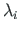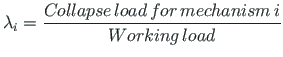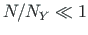# 7.1 Introduction

An analysis of a structure is an important means for estimating its serviceability under the loading for which the structure has been designed. If the load is increased until the structure reaches a full yield, plastic hinges will form and the structure will transform to a mechanism. Under further loading this mechanism would collapse. The number of plastic hinges required to convert a structure into a mechanism is one more than the degree of indeterminacy (n+1). A study of the collapse load magnitude is necessary to determine the load factor.[A typical stress-strain curve for mild steel][An idealized stress-strain curve]

The load factor[Cpr11] for a collapse mechanism i is(7.1)

where the collapse load is the load at which the structure will fail, and the working load is the load which the structure is expected to carry.

In statically indeterminate structures the collapse load is larger than the load that initiates yielding.

Let us consider an ideal elastic-plastic material yielding (see Prandtl7.1 diagram Fig. 7.1(b)) in the beam cross section.

In structural members subjected to bending, an assumption that a plastic hinge might form at a point is sufficient for limit state analysis. At a plastic hinge, stresses remain constant (see Fig. 7.2), whereas strains and rotations can increase. We assume that the condition for the axial forceis fulfilled, otherwise plastic bending and compression or tension must be combined [IB01].

Subsections
andres
2014-09-09